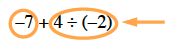### Home > CC2 > Chapter 3 > Lesson 3.2.5 > Problem3-83

3-83.

Follow the Order of Operations (use circling the terms if it is helpful) to simplify the following expressions: Homework Help ✎

1. $- 7 + 4 \div ( - 2 )$

If you need help with simplifying expressions, refer to earlier problems on the Order of Operations. Try working it out on your own before checking the answer.$-9$

1. $17.5 \div ( - 7 ) + - 8.1 ( 2 )$

Refer to part (a) if you are having trouble getting started. Remember to divide and multiply before adding or subtracting!

$-18.7$

1. $( 8 + ( - 3 ) ) ( - 5 \frac { 3 } { 5 } )$

This problem is similar to the previous two. Pay extra attention to parentheses!

Sophia thinks that the answer is $23$, because multiplication should come before addition.
Do you agree with her? Is there any reason that you might need to add first in this problem?

1. $( 4 - ( - 3 ) ) + ( 5 \div ( - 5 ) )$

This problem is similar to the previous three. Be careful to follow the Order of Operations as you calculate.